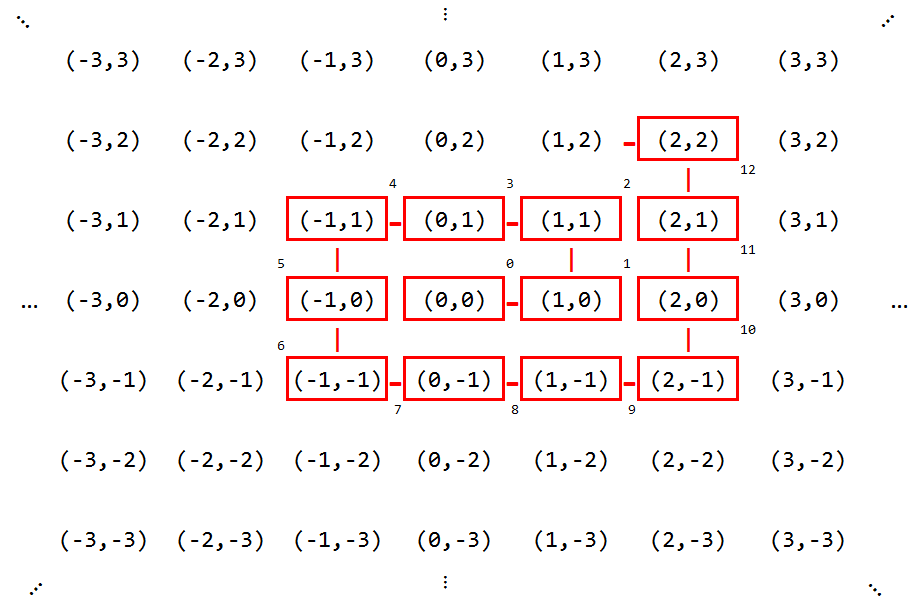# Solution to a puzzle

Nov 20, 2012

Last time, I presented a mathematical puzzle.  Here, I will explain a solution.  If you have  come across this page first, but don’t want the solution spoiled, stop reading now!

## The Solution

Start by defining the spatial and temporal coordinates. Take any arbitrary point in our 1-dimensional discrete space and label it $$x = 0$$ .  For time, define $$t = 0$$ as the time at which we fire our first torpedo.

We don’t know the position or velocity of the ship at $$t = 0$$ , but we do know that these two numbers completely define any potential ship’s trajectory for all future time.  Thus we can label any possible ship’s trajectory $$S$$ with a tuple $$(X, V)$$ , where $$X$$ is the ship’s position at $$t = 0$$ , and $$V$$ is the ship’s velocity.  Per the problem statement, $$X$$ and $$V$$ are both integers.

Given a particular $$S = (X, V)$$ , it is simple to calculate where the ship will be at any particular time.  For example, if $$S = (10, 6)$$ , then at $$t = 20$$ the ship will be at position $$x = 130$$ .

There is an infinite number of possible starting positions, and an infinite number of velocities, so clearly there is an infinite number of possible trajectories.  The key insight is that although their number is infinite, the trajectories can still be arranged into a single (infinitely long) list.  That is, the set of potential trajectories is countably infinite.  I’ll go into how to construct this list later.

If we can make a list of all potential ship’s trajectories, then we can immediately create a torpedo detonation strategy:  At time $$t = i$$ , fire a torpedo at the position where the ship would be if it were following the $$i^{\text{th}}$$ trajectory in the list.

Using this strategy, a ship traveling along any possible trajectory will eventually be hit – it’s simply a matter of how long that will take.  How long it will take is governed solely by where that trajectory winds up in the list.  Although the list itself is infinitely long, any given element of the list must still be at some finite index.  Therefore we have met the requirements of the puzzle - we have an algorithm for torpedo detonation which will find any single ship within a finite amount of time.

### How to list the trajectories

The above relies on our theoretical ability to create an indexed list of all possible trajectories.  How do we actually do that?

One way (the way many are taught in undergrad math courses) is to arrange the trajectory tuples in an infinite grid, where the “center” is the tuple (0,0), and select elements in a spiral, numbering as we go.  From the diagram below it’s easy to convince yourself that every possible tuple will be included somewhere in the list.Using this list of trajectories, our first few torpedo shots (corresponding to the boxed trajectories in the diagram) would be at positions 0, 1, 3, 3, 3, -1, -7, -7, -7, -7, 2, 13, 24.